# Help with disappeared column

Member Posts: 10Newbie
Good morning,
I am trying to use several join operators to be able to make 1 to n relationships from several tables created previously.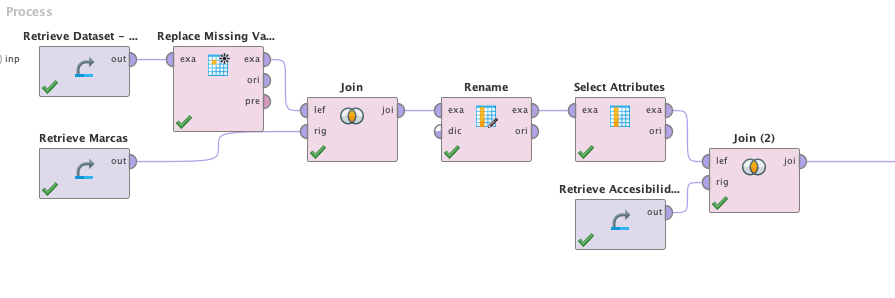That join seems to have only the new table Accessibility and the previously selected attributes.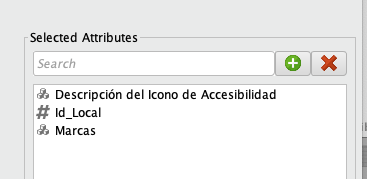The weird thing is that I have not selected Id_Marcas, anywhere and it appears alone.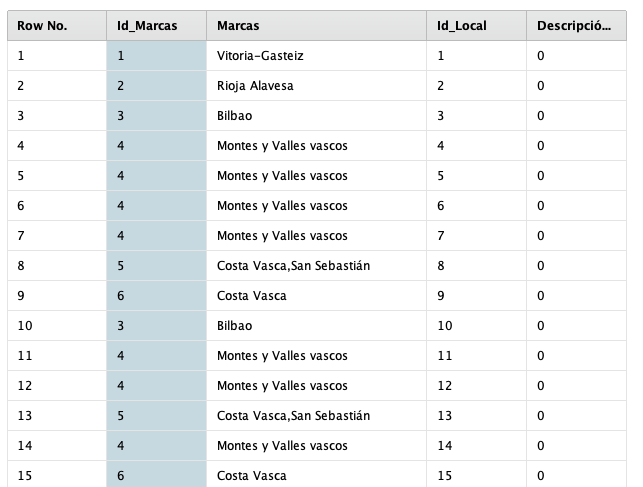Where I have the box Rename, I have renamed id to id_marcas, as I am going to do many joins afterward. I don't get the point of reusing the column as there are different joins with different attributes and tables.

Thank you

Tagged:

• Member Posts: 473Unicorn
edited April 2021
Hi, Egun on @OierMk,

I recommend you to verify the output of Operators using a breakpoint after.
Can you share your process in order to reproduce the error?

Best
• Member Posts: 10Newbie
Aupa,

these are the images of the process with the breakpoints.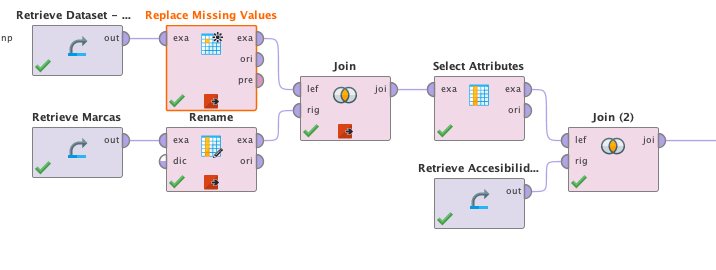Original process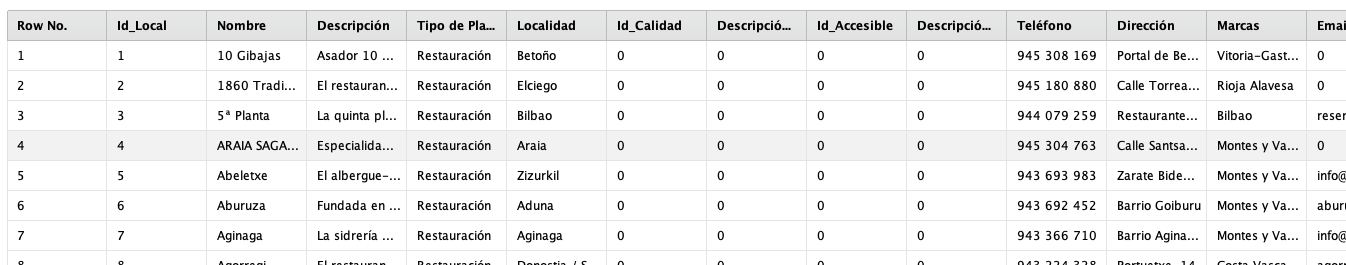After the replacing the null or inexistent values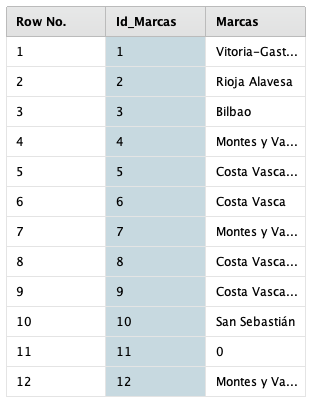The "marcas" table after "Id" column changes its name to "Id_Marcas"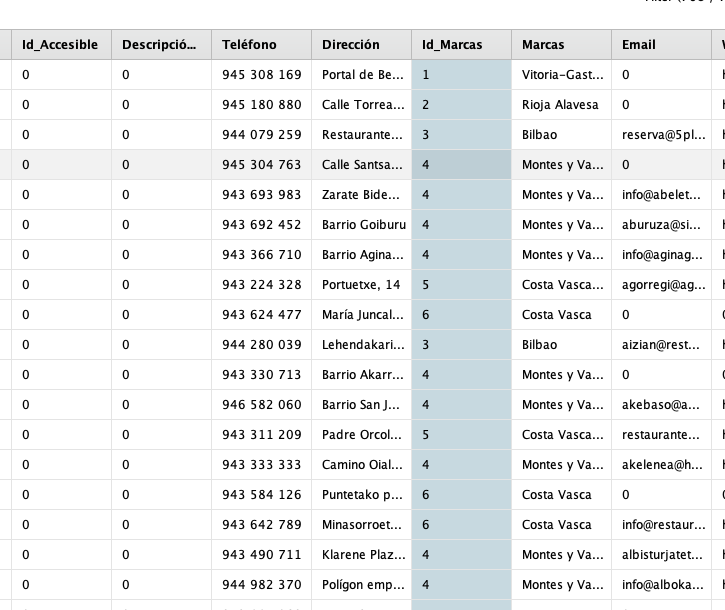The result of the first join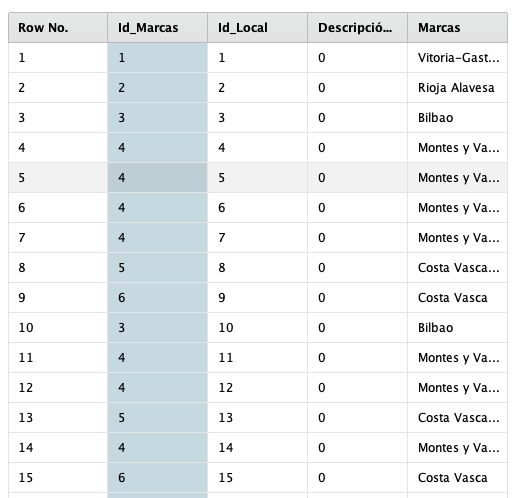Result after selecting the valid attributes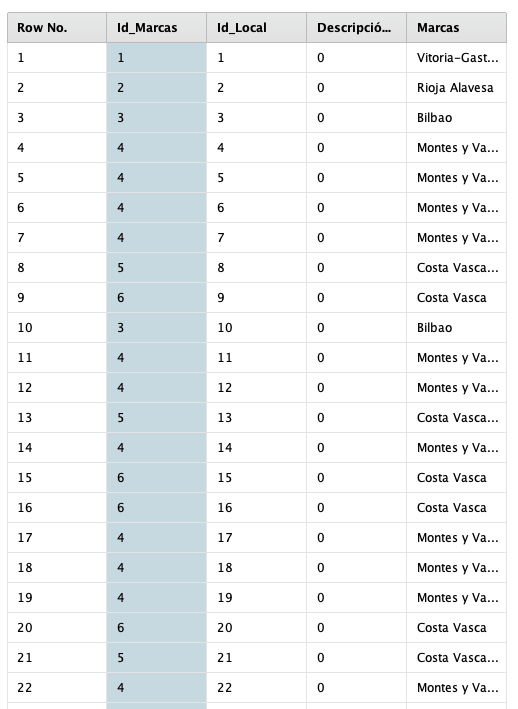The result after the 2nd join

Where has the id of accessibility gone?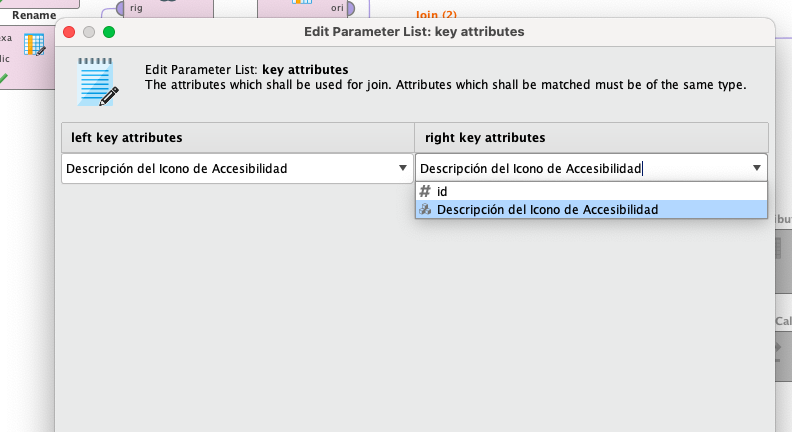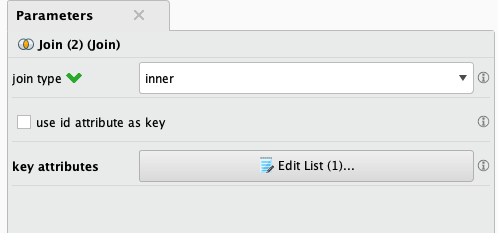Composition of the join

Thank you soo much
• Member Posts: 473Unicorn
Aupa @OierMk,

How to Post Questions on the RapidMiner User Community — RapidMiner Community

for the pictures I suppose that your Attributes are not the same type.
Check if both attributes are real, or polynominal, etc.

Best
• Member Posts: 10Newbie
Oh sorry, it has been my first post, I didn't know it. I think that all attributes are the same type.

I leave the code here

<?xml version="1.0" encoding="UTF-8"?>
• Member Posts: 10Newbie
```<?xml version="1.0" encoding="UTF-8"?><process version="9.9.000">
<context>
<input/>
<output/>
<macros/>
</context>
<operator activated="true" class="process" compatibility="9.9.000" expanded="true" name="Process">
<parameter key="logverbosity" value="init"/>
<parameter key="random_seed" value="2001"/>
<parameter key="send_mail" value="never"/>
<parameter key="process_duration_for_mail" value="30"/>
<parameter key="encoding" value="SYSTEM"/>
<process expanded="true">
<operator activated="true" class="retrieve" compatibility="9.9.000" expanded="true" height="68" name="Retrieve Marcas" width="90" x="45" y="136">
<parameter key="repository_entry" value="Marcas"/>
</operator>
<operator activated="true" class="retrieve" compatibility="9.9.000" expanded="true" height="68" name="Retrieve Dataset - ETL" width="90" x="45" y="34">
<parameter key="repository_entry" value="Dataset - ETL"/>
</operator>
<operator activated="true" class="replace_missing_values" compatibility="9.9.000" expanded="true" height="103" name="Replace Missing Values" width="90" x="179" y="34">
<parameter key="return_preprocessing_model" value="false"/>
<parameter key="create_view" value="false"/>
<parameter key="attribute_filter_type" value="all"/>
<parameter key="attribute" value="Descripción del Icono de Accesibilidad"/>
<parameter key="attributes" value=""/>
<parameter key="use_except_expression" value="false"/>
<parameter key="value_type" value="attribute_value"/>
<parameter key="use_value_type_exception" value="false"/>
<parameter key="except_value_type" value="time"/>
<parameter key="block_type" value="attribute_block"/>
<parameter key="use_block_type_exception" value="false"/>
<parameter key="except_block_type" value="value_matrix_row_start"/>
<parameter key="invert_selection" value="false"/>
<parameter key="include_special_attributes" value="true"/>
<parameter key="default" value="value"/>
<list key="columns"/>
<parameter key="replenishment_value" value="0"/>
</operator>
<operator activated="true" class="retrieve" compatibility="9.9.000" expanded="true" height="68" name="Retrieve Accesibilidad" width="90" x="447" y="187">
</operator>
<operator activated="false" class="retrieve" compatibility="9.9.000" expanded="true" height="68" name="Retrieve Calidad" width="90" x="916" y="442">
</operator>
<operator activated="false" class="retrieve" compatibility="9.9.000" expanded="true" height="68" name="Retrieve Capacidad" width="90" x="1050" y="493">
</operator>
<operator activated="false" class="retrieve" compatibility="9.9.000" expanded="true" height="68" name="Retrieve CPostal" width="90" x="1184" y="544">
<parameter key="repository_entry" value="CPostal"/>
</operator>
<operator activated="false" class="retrieve" compatibility="9.9.000" expanded="true" height="68" name="Retrieve Municipio" width="90" x="1318" y="595">
<parameter key="repository_entry" value="Municipio"/>
</operator>
<operator activated="false" class="retrieve" compatibility="9.9.000" expanded="true" height="68" name="Retrieve Plantilla" width="90" x="1452" y="646">
<parameter key="repository_entry" value="Plantilla"/>
</operator>
<operator activated="false" class="retrieve" compatibility="9.9.000" expanded="true" height="68" name="Retrieve Tipo" width="90" x="1586" y="697">
<parameter key="repository_entry" value="Tipo"/>
</operator>
<operator activated="true" class="blending:rename" compatibility="9.9.000" expanded="true" height="82" name="Rename" width="90" x="179" y="136">
<list key="rename attributes">
<parameter key="id" value="Id_Marcas"/>
</list>
<parameter key="from_attribute" value=""/>
<parameter key="to_attribute" value=""/>
</operator>
<operator activated="true" class="concurrency:join" compatibility="9.9.000" expanded="true" height="82" name="Join" width="90" x="313" y="85">
<parameter key="remove_double_attributes" value="true"/>
<parameter key="join_type" value="inner"/>
<parameter key="use_id_attribute_as_key" value="false"/>
<list key="key_attributes">
<parameter key="Marcas" value="Marcas"/>
</list>
<parameter key="keep_both_join_attributes" value="false"/>
</operator>
<operator activated="true" breakpoints="after" class="select_attributes" compatibility="9.9.000" expanded="true" height="82" name="Select Attributes" width="90" x="447" y="85">
<parameter key="attribute_filter_type" value="subset"/>
<parameter key="attribute" value=""/>
<parameter key="attributes" value="Id_Local|Id_Marcas|Marcas|Descripción del Icono de Accesibilidad"/>
<parameter key="use_except_expression" value="false"/>
<parameter key="value_type" value="attribute_value"/>
<parameter key="use_value_type_exception" value="false"/>
<parameter key="except_value_type" value="time"/>
<parameter key="block_type" value="attribute_block"/>
<parameter key="use_block_type_exception" value="false"/>
<parameter key="except_block_type" value="value_matrix_row_start"/>
<parameter key="invert_selection" value="false"/>
<parameter key="include_special_attributes" value="false"/>
</operator>
<operator activated="true" breakpoints="after" class="concurrency:join" compatibility="9.9.000" expanded="true" height="82" name="Join (2)" width="90" x="581" y="136">
<parameter key="remove_double_attributes" value="true"/>
<parameter key="join_type" value="inner"/>
<parameter key="use_id_attribute_as_key" value="false"/>
<list key="key_attributes">
</list>
<parameter key="keep_both_join_attributes" value="false"/>
</operator>
<operator activated="false" breakpoints="before" class="blending:rename" compatibility="9.9.000" expanded="true" height="82" name="Rename (2)" width="90" x="782" y="340">
<list key="rename attributes">
</list>
<parameter key="from_attribute" value=""/>
<parameter key="to_attribute" value=""/>
</operator>
<operator activated="false" class="select_attributes" compatibility="9.9.000" expanded="true" height="82" name="Select Attributes (2)" width="90" x="916" y="340">
<parameter key="attribute_filter_type" value="subset"/>
<parameter key="attribute" value=""/>
<parameter key="use_except_expression" value="false"/>
<parameter key="value_type" value="attribute_value"/>
<parameter key="use_value_type_exception" value="false"/>
<parameter key="except_value_type" value="time"/>
<parameter key="block_type" value="attribute_block"/>
<parameter key="use_block_type_exception" value="false"/>
<parameter key="except_block_type" value="value_matrix_row_start"/>
<parameter key="invert_selection" value="false"/>
<parameter key="include_special_attributes" value="false"/>
</operator>
<operator activated="false" breakpoints="after" class="concurrency:join" compatibility="9.9.000" expanded="true" height="82" name="Join (3)" width="90" x="1050" y="391">
<parameter key="remove_double_attributes" value="true"/>
<parameter key="join_type" value="left"/>
<parameter key="use_id_attribute_as_key" value="false"/>
<list key="key_attributes">
</list>
<parameter key="keep_both_join_attributes" value="false"/>
</operator>
<operator activated="false" class="concurrency:join" compatibility="9.9.000" expanded="true" height="82" name="Join (4)" width="90" x="1184" y="442">
<parameter key="remove_double_attributes" value="true"/>
<parameter key="join_type" value="left"/>
<parameter key="use_id_attribute_as_key" value="false"/>
<list key="key_attributes">
</list>
<parameter key="keep_both_join_attributes" value="false"/>
</operator>
<operator activated="false" class="concurrency:join" compatibility="9.9.000" expanded="true" height="82" name="Join (5)" width="90" x="1318" y="493">
<parameter key="remove_double_attributes" value="true"/>
<parameter key="join_type" value="left"/>
<parameter key="use_id_attribute_as_key" value="false"/>
<list key="key_attributes">
<parameter key="Postal Code" value="Postal Code"/>
</list>
<parameter key="keep_both_join_attributes" value="false"/>
</operator>
<operator activated="false" class="concurrency:join" compatibility="9.9.000" expanded="true" height="82" name="Join (6)" width="90" x="1452" y="544">
<parameter key="remove_double_attributes" value="true"/>
<parameter key="join_type" value="left"/>
<parameter key="use_id_attribute_as_key" value="false"/>
<list key="key_attributes">
<parameter key="Municipio" value="Municipio"/>
</list>
<parameter key="keep_both_join_attributes" value="false"/>
</operator>
<operator activated="false" class="concurrency:join" compatibility="9.9.000" expanded="true" height="82" name="Join (7)" width="90" x="1586" y="595">
<parameter key="remove_double_attributes" value="true"/>
<parameter key="join_type" value="left"/>
<parameter key="use_id_attribute_as_key" value="false"/>
<list key="key_attributes">
<parameter key="Tipo de Plantilla" value="Tipo de Plantilla"/>
</list>
<parameter key="keep_both_join_attributes" value="false"/>
</operator>
<operator activated="false" class="concurrency:join" compatibility="9.9.000" expanded="true" height="82" name="Join (8)" width="90" x="1720" y="646">
<parameter key="remove_double_attributes" value="true"/>
<parameter key="join_type" value="left"/>
<parameter key="use_id_attribute_as_key" value="false"/>
<list key="key_attributes">
<parameter key="Tipo de Restauración" value="Tipo de Restauración"/>
</list>
<parameter key="keep_both_join_attributes" value="false"/>
</operator>
<connect from_op="Retrieve Marcas" from_port="output" to_op="Rename" to_port="example set input"/>
<connect from_op="Retrieve Dataset - ETL" from_port="output" to_op="Replace Missing Values" to_port="example set input"/>
<connect from_op="Replace Missing Values" from_port="example set output" to_op="Join" to_port="left"/>
<connect from_op="Retrieve Accesibilidad" from_port="output" to_op="Join (2)" to_port="right"/>
<connect from_op="Retrieve Calidad" from_port="output" to_op="Join (3)" to_port="right"/>
<connect from_op="Retrieve Capacidad" from_port="output" to_op="Join (4)" to_port="right"/>
<connect from_op="Retrieve CPostal" from_port="output" to_op="Join (5)" to_port="right"/>
<connect from_op="Retrieve Municipio" from_port="output" to_op="Join (6)" to_port="right"/>
<connect from_op="Retrieve Plantilla" from_port="output" to_op="Join (7)" to_port="right"/>
<connect from_op="Retrieve Tipo" from_port="output" to_op="Join (8)" to_port="right"/>
<connect from_op="Rename" from_port="example set output" to_op="Join" to_port="right"/>
<connect from_op="Join" from_port="join" to_op="Select Attributes" to_port="example set input"/>
<connect from_op="Select Attributes" from_port="example set output" to_op="Join (2)" to_port="left"/>
<connect from_op="Join (2)" from_port="join" to_port="result 1"/>
<connect from_op="Rename (2)" from_port="example set output" to_op="Select Attributes (2)" to_port="example set input"/>
<connect from_op="Select Attributes (2)" from_port="example set output" to_op="Join (3)" to_port="left"/>
<connect from_op="Join (3)" from_port="join" to_op="Join (4)" to_port="left"/>
<connect from_op="Join (4)" from_port="join" to_op="Join (5)" to_port="left"/>
<connect from_op="Join (5)" from_port="join" to_op="Join (6)" to_port="left"/>
<connect from_op="Join (6)" from_port="join" to_op="Join (7)" to_port="left"/>
<connect from_op="Join (7)" from_port="join" to_op="Join (8)" to_port="left"/>
<portSpacing port="source_input 1" spacing="0"/>
<portSpacing port="sink_result 1" spacing="0"/>
<portSpacing port="sink_result 2" spacing="0"/>
</process>
</operator>
</process>```

• Member Posts: 473Unicorn
Well done @OierMk
Can you share a sample of your datasets?
Best

• Member Posts: 10Newbie
• Member Posts: 473Unicorn
edited April 2021
Hi @OierMk
Please remove the "label" role to the id_Marcas Attribute. in some place you change the role of this attribute.
That's the why it appear, even if you don't select it.

Best
• Member Posts: 10Newbie
Hi @ceaperez I know what you mean, but doing so, I will have only 1 column with the id of every join, i would be rewriting the same column instead of creating new ones in each join, which is what i want.

• Member Posts: 473Unicorn
Hi @OierMk
Now i'm confused. that is not the original question. If you want to preserve both attributes in in a join operator you need to check the option "keep the both join attributes" That's what you need?

Best
• Member Posts: 10Newbie
I want to do a simple join as in sql, I am trying to normalize the data until I get the 3rd normal form. To do so, I am taking all the data from excel and I have splitted all the possible tables following the rules. Now, I am trying to link all the data of the different tables into the main table with all the attributes. For so I need to get all the primary keys, the ones I am trying to add to the main table with the joins.

Did I make myself clearer?

Thank you for your time @ceaperez sorry for the misunderstanding.
• Member Posts: 473Unicorn
Hi @OierMk

Don't worry. I'm here to help.
You are almost there. In your datasets you don't have any attribute named id accesibilidad.

Best

• Member Posts: 10Newbie
Hi @ceaperez

I know, i have an attribute called id. Even so, i change the name with a box called rename attribute to try that way not to overwrite the data i have. The point is that i don't know what is happening because the join doesn't give me the new id joined at the second join.
• Member Posts: 473Unicorn
Aupa @OierMk

Something like this?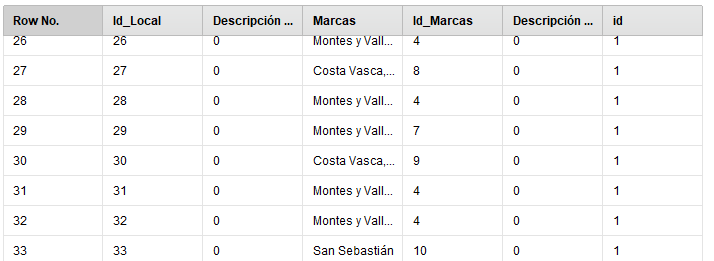• Member Posts: 10Newbie
That's exactly what I need! How did you get it @ceaperez?
• Member Posts: 473Unicorn
Hi @OierMk

• Member Posts: 10Newbie